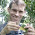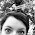## Wednesday, September 18, 2013

### Coloring tip labels by character state

"I want to color my tree tip labels according to a particular character and provide a legend for the same."

Here's a demo of one way to do this, using the phytools data object anoletree:

require(phytools)
data(anoletree)
tree<-anoletree
x<-getStates(tree,"tips")
par(fg="transparent")
plotTree(tree,fsize=0.6,ylim=c(-1,length(tree\$tip.label)))
lastPP<-get("last_plot.phylo",env=.PlotPhyloEnv)
ss<-sort(unique(x))
colors<-setNames(palette()[1:length(ss)],ss)
par(fg="black")
y=-1,prompt=FALSE)
colors<-sapply(x,function(x,y) y[which(names(y)==x)],
y=colors)
tt<-gsub("_"," ",tree\$tip.label)
text(lastPP\$xx[1:length(tt)],lastPP\$yy[1:length(tt)],
tt,cex=0.6,col=colors,pos=4,offset=0.1,font=3)

Here's the result:

Obviously, some of this is idiosyncratic to this specific dataset. Hopefully, users can figure out how to modify this to their own data and problem.

1.Thanks for this code! I have binary states and can't quite seem to tweak the code right. I can assign the colors (black or red) based on the binary state, but when I try to write the text to the figure the colors come out jumbled. Thoughts?

2.If you have a vector colors with the colors you want for the tips, and names(colors) equal to the tip names sort by the vector tip.label & try:

colors<-colors[tree\$tip.label]

Let us know if that does the trick. Liam

3.I have a question for you. The part of the code

lastPP<-get("last_plot.phylo",env=.PlotPhyloEnv)
tt<-gsub("_"," ",tree\$tip.label)
text(lastPP\$xx[1:length(tt)],lastPP\$yy[1:length(tt)],
tt,cex=0.6,col=colors,pos=4,offset=0.1,font=3)

can help me to extract the x and y coordinates where the tips are located?
I want to plot some boxplots on the tips of the tree but the location of them has proven to be difficult, so I was wondering if this will help me align the boxplots to the tips.

4.Hi Liam,
Do you have a suggestion for changing the tip colors to correspond to a discrete character (as in the example above) in the function phenogram, while preserving the spread.labels?
Thanks!

Note: due to the very large amount of spam, all comments are now automatically submitted for moderation.